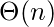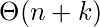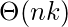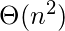# Exercise: Questions on Queue

Questions on Queue : Question 1 :
Following is C like pseudo code of a function that takes a Queue as an argument, and uses a stack S to do processing.
```void fun(Queue *Q)
{
Stack S;  // Say it creates an empty stack S

// Run while Q is not empty
while (!isEmpty(Q))
{
// deQueue an item from Q and push the dequeued item to S
push(&S, deQueue(Q));
}

// Run while Stack S is not empty
while (!isEmpty(&S))
{
// Pop an item from S and enqueue the poppped item to Q
enQueue(Q, pop(&S));
}
}
```
What does the above function do in general?

 Removes the last from Q Keeps the Q same as it was before the call Makes Q empty Reverses the Q  Correct
Questions on Queue : Question 2 :
Which one of the following is an application of Queue Data Structure?

 When a resource is shared among multiple consumers. When data is transferred asynchronously (data not necessarily received at same rate as sent) between two processes Load Balancing All of the above  Correct
Questions on Queue : Question 3 :
How many stacks are needed to implement a queue. Consider the situation where no other data structure like arrays, linked list is available to you.

 1 2  Correct 3 4
Questions on Queue : Question 4 :
How many queues are needed to implement a stack. Consider the situation where no other data structure like arrays, linked list is available to you.

 1 2  Correct 3 4
Questions on Queue : Question 5 :
A priority queue can efficiently implemented using which of the following data structures? Assume that the number of insert and peek (operation to see the current highest priority item) and extraction (remove the highest priority item) operations are almost same.

 Array Linked List Heap Data Structures like Binary Heap, Fibonacci Heap  Correct None of the above
Questions on Queue : Question 6 :
Which of the following is true about linked list implementation of queue?

 In push operation, if new nodes are inserted at the beginning of linked list, then in pop operation, nodes must be removed from end. In push operation, if new nodes are inserted at the end, then in pop operation, nodes must be removed from the beginning. Both of the above  Correct None of the above
Questions on Queue : Question 7 :
Suppose a circular queue of capacity (n â 1) elements is implemented with an array of n elements. Assume that the insertion and deletion operation are carried out using REAR and FRONT as array index variables, respectively. Initially, REAR = FRONT = 0. The conditions to detect queue full and queue empty are

 Full: (REAR+1) mod n == FRONT, empty: REAR == FRONT  Correct Full: (REAR+1) mod n == FRONT, empty: (FRONT+1) mod n == REAR Full: REAR == FRONT, empty: (REAR+1) mod n == FRONT Full: (FRONT+1) mod n == REAR, empty: REAR == FRONT
Questions on Queue : Question 8 :
A Priority-Queue is implemented as a Max-Heap. Initially, it has 5 elements. The level-order traversal of the heap is given below: 10, 8, 5, 3, 2 Two new elements ”1‘ and ”7‘ are inserted in the heap in that order. The level-order traversal of the heap after the insertion of the elements is:

 10, 8, 7, 5, 3, 2, 1 10, 8, 7, 2, 3, 1, 5 10, 8, 7, 1, 2, 3, 5 10, 8, 7, 3, 2, 1, 5  Correct
Questions on Queue : Question 9 :
An implementation of a queue Q, using two stacks S1 and S2, is given below:
```void insert(Q, x) {
push (S1, x);
}

void delete(Q){
if(stack-empty(S2)) then
if(stack-empty(S1)) then {
print(âQ is emptyâ);
return;
}
else while (!(stack-empty(S1))){
x=pop(S1);
push(S2,x);
}
x=pop(S2);
}
```
Let n insert and m (<=n) delete operations be performed in an arbitrary order on an empty queue Q. Let x and y be the number of push and pop operations performed respectively in the process. Which one of the following is true for all m and n?

 n+m <= x < 2n and 2m <= y <= n+m  Correct n+m <= x < 2n and 2m<= y <= 2n 2m <= x < 2n and 2m <= y <= n+m 2m <= x <2n and 2m <= y <= 2n
Questions on Queue : Question 10 :
Consider the following operation along with Enqueue and Dequeue operations on queues, where k is a global parameter.
```MultiDequeue(Q){
m = k
while (Q is not empty and m  > 0) {
Dequeue(Q)
m = m - 1
}
}```
What is the worst case time complexity of a sequence of n MultiDequeue() operations on an initially empty queue? (GATE CS 2013) (A)(B)(C)(D)A  Correct B C D